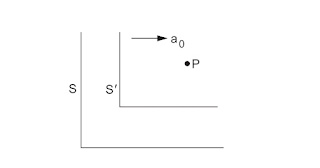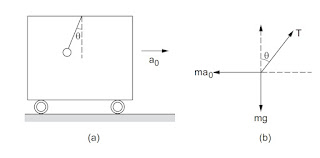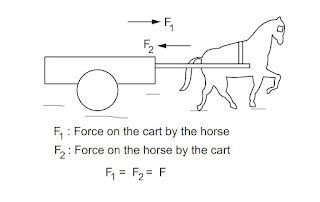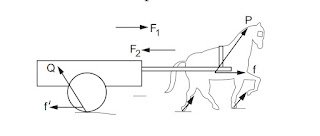# Pseudo force definition

Pseudo forces are the techniques of solving the motion of a body concerning a non-inertial frame of reference.

Pseudo force is also called fictitious force.

# Pseudo force formula

The formula for pseudo or fictitious force is

ma = F - ma0

Where a = acceleration

a0 = constant acceleration

m = mass of the body

F = applied force

# Explaining pseudo or fictitious force with examples

Consider the situation shown in the given figure. Suppose the frame of reference S' moves with a constant acceleration a0 with respect to an inertial frame S. The acceleration of a particle P measured with respect to S' is AP, S' = a, and that with respect to S is Ap,s. The acceleration of S' with respect to S is as',s = a0.Since S ' is translating with respect to S we have,

ap,s' = ap,s + as,s' = ap,s - as',s

or,

a = ap,s - a0

or,

m a = m ap,s - m a0

where m is the mass of the particle P. Since S is an inertial frame m ap,s is equal to the sum of all the forces acting on P. Writing this sum as F, we get

m a = F - m a0

or,

a = F-ma0/ m ........ (5.3)

This equation relates the acceleration of the particle and the forces acting on it. Compare it with the equation which relates the acceleration and the force when the acceleration is measured concerning an inertial frame. The acceleration of the frame (with respect to an inertial frame) comes into the equation of a particle.

Newton's second law a = F / m is not valid in such a non-inertial frame. An extra term - m a0, has to be added to the sum of all the forces acting on the particle before writing the equation a = F/m.

Note that in this extra term m is the mass of the particle under consideration and a0 is the acceleration of the working frame of reference with respect to some inertial frame.

However, we people spend most of our lifetime on the earth which is an (approximate) inertial frame. We are so familiar with Newton's laws that we would still like to use the terminology of Newton's laws even when we use a noninertial frame. This can be done if we agree to call ( - ma0. ) a force acting on the particle. Then while preparing the list of forces acting on the particle P, we include all the ( real ) forces acting on P by all other objects and also include an imaginary force - m a0.

Applying Newton's second law will then lead to equation ( 5.3 ). Such correction terms - ma, in the list of forces are called pseudo forces. This so-called force is to be included in the list only because we are discussing the motion from a noninertial frame and still want to use Newton's second law as " total force equals mass times acceleration ".

If we work from an inertial frame, the acceleration a0, of the frame is zero and no pseudo force is needed. The pseudo forces are also called inertial forces although their need arises because of the use of noninertial frames.

Example of pseudo force

A pendulum is hanging from the ceiling of a car having an acceleration a0, with respect to the road. Find the angle made by the string with the vertical.

Solution:

The situation is shown in the given figure. Suppose the mass of the bob is m and the string makes an angle o with the vertical We shall work from the car frame. This frame is noninertial as it has an acceleration a0, concerning an inertial frame ( the road). Hence, if we use Newton's second law we shall have to include a pseudo force.Take the bob as the system.

The forces are:

1. ( a ) T along the string, by the string
2. ( b ) mg downward, by the earth
3. ( c ) ma, towards left ( pseudo force ).

The free-body diagram is shown in the figure. As the bob is at rest (remember we are discussing the motion with respect to the car) the force in (a), (b), and (c) should add to zero. Take the X-axis along the forward horizontal direction and Y-axis along the upward vertical direction. The components of the forces along with the X-axis give

T sino - m a0 = 0 or , T sino = m a0, .........(i)

and the components along the Y-axis give

T coso - mg = 0 or T coso = mg .............(ii)

Dividing ( i ) by ( ii ) tano = a0 / g .

Thus, the string makes an angle tan-1 ( a0 / g ) with the vertical.

# The horse and the cart problem with proper diagram

A good example which illustrates the ideas discussed is the motion of a cart pulled by a horse. Suppose the cart is at rest when the driver whips the horse. The horse pulls the cart and the cart accelerates forward. The question posed is as follows.

The horse pulls the cart by a force F1, in the forward direction. From the third law of motion, the cart pulls the horse by an equal force F1, = F2, in the backward direction. The sum of these forces is, therefore, zero. Why should then the cart accelerate forward?

Try to locate the mistake in the argument. According to our scheme, we should first decide the system. We can take the horse as the system or the cart as the system or the cart and the horse taken together as the system. Suppose you take the cart as the system. Then the forces on the cart should be listed and the forces on the horse should not enter the discussion.The force on the cart is F1, in the forward direction and the acceleration of the cart is also in the forward direction. How much is this acceleration? Take the mass of the cart to be Mc. Is the acceleration of the cart a = F1 / Mc in the forward direction? Think carefully. We shall return to this question.

Let us now try to understand the motion of the horse. This time we have to consider the forces on the horse. The forward force F1, by the horse, acts on the cart and it should not be taken into account when we discuss the motion of the horse. The force on the horse by the cart is F2, in the backward direction.

Why does the horse go in the forward direction when whipped?

The horse exerts a force on the cart in the forward direction and hence the cart is accelerated forward. But the cart exerts an equal force on the horse in the backward direction.

Why is the horse not accelerated in a backward direction? ( Imagine this situation. If the cart is accelerated forward and the horse backward, the horse will sit on the cart kicking out the driver and the passenger. ) Where are we wrong?

We have not considered all the forces acting on the horse. The road pushes the horse by a force P which has a forward component. This force acts on the horse and we must add this force when we discuss the motion of the horse.

The horse accelerates forward if the forward component f of the force P exceeds F2. The acceleration of the horse is ( f - F2 ) / Mh. We should make sure that all the forces acting on the system are added. Note that the force of gravity acting on the horse has no forward component.Going back to the previous paragraph the acceleration of the cart may not be F1 / Mc. The road exerts a force Q on the cart which may have a backward component f '. The total force on the cart is F1 - f '. The acceleration of the cart is then a = F - f ' / Mc in the forward direction. The forces f and f ' are self adjustable and they so adjust their values [ F1 - f '/ Mc ] = [ f - F2 / Mh ].

The acceleration of the horse and that of the cart are equal in magnitude and direction and hence they move together. So, once again we remind you that only the forces on the system are to be considered to discuss the motion of the system and all the forces acting on the system are to be considered. Only then apply F = m a.